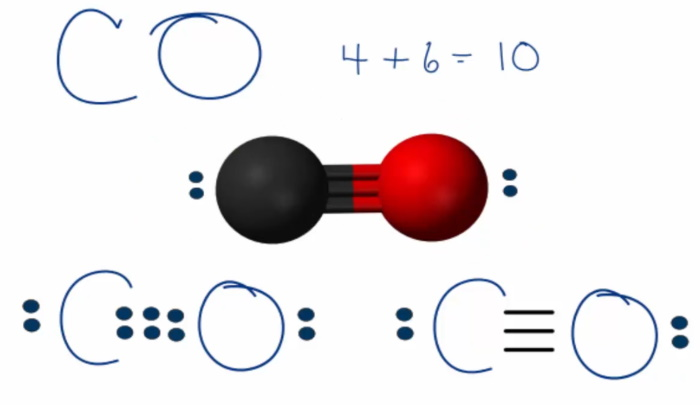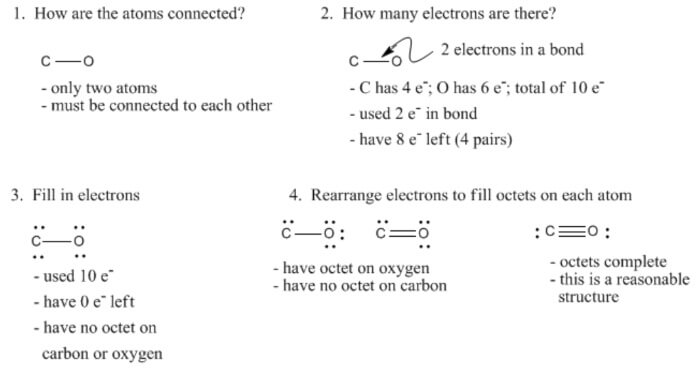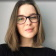# Draw the Lewis structure for CO

I need to draw the Lewis structure for CO.

What is the Lewis structure of CO?

How to draw the Lewis dot diagram for Carbon monoxide (CO)?

﻿
1. A step-by-step explanation of how to draw the Lewis Dot Diagram for CO.

Use the periodic table of elements to find the total number of valence electrons for the CO molecule. Once we know how many valence electrons does carbon monoxide have we can distribute them around the central atom with the goal of filling the outer shells of each atom.

You should to find how many electrons does carbon monoxide have, how many each atom needs, how many of those are bonding, and how many are lone pairs. This data can then be used to determine the Lewis Dot Structure.

1) Find valence e for all atoms. Add them together.

C: 4
O: 6

Total = 10

2) Find octet e for each atom and add them together.

C: 8
O: 8

Total = 16

3) Find the bonding e. Subtract step 1 total from step 2

16-10 = 6e

4) Find number of bonds by dividing the number of bonding electrons by 2 (because each bond is made of 2 e)

6e/2 = 3 bonds

5) The rest are nonbonding pairs. Subtract bonding electrons (step 3) from valence electrons (step 1).

10-6 = 4e = 2 lone pairs

Use information from step 4 and 5 to draw the CO.

CO Lewis Structure:1. The Lewis Structure for CO:

1. Count the electrons.
2. Put least electronegative atom in centre.
3. Put one electron pair in each bond.
4. Fill outer atoms with electrons.
5. Move electrons so all atoms has a full octet.

1. ### A Strategy For Constructing Lewis Structures: Example With Carbon Monoxide, COPsst... Stuck with your assignment? 😱Erica
OnlinePsst... Stuck with your assignment? 😱
Do you need an essay to be done?
Yes
Other assignmentWhat type of assignment 📝 do you need?
• Article review
• Case study
• Coursework
• Report
• Research Paper
• Other AssignmentHow many pages (words) do you need? Let's see if we can help you!